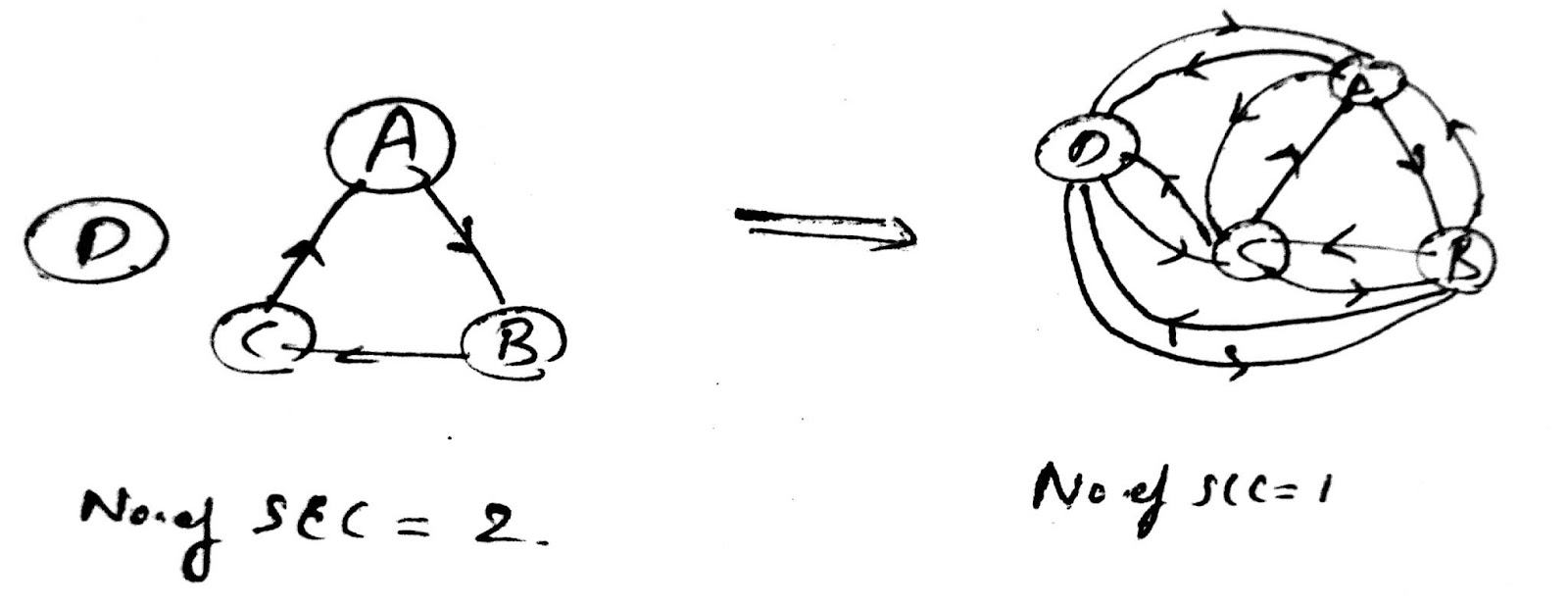# GATE2014-1-3

5.9k views

Let $G=(V,E)$ be a directed graph where $V$ is the set of vertices and $E$ the set of edges. Then which one of the following graphs has the same strongly connected components as $G$ ?

1. $G_1$ = $(V,E_1)$ where $E_1 = \left\{(u,v) \mid (u,v) \notin E\right\}$
2. $G_2$ = $(V,E_2)$ where $E_2 = \left\{(u,v) \mid (v,u) \in E \right\}$
3. $G_3$ = $(V,E_3)$ where $E_3 = \{(u,v) \mid$ there is a path of length $\leq2$ from $u$ to $v$ in $E\}$
4. $G_4$ = $(V_4,E)$ where $V_4$ is the set of vertices in $G$ which are not isolated
in DS
recategorized
4
Both B & C seem true.
00
Updated ..that was a typo
0
What is strongly connected component?
1

strongly connected component means, if there is a path exists between all the vertices of the component

https://www.geeksforgeeks.org/strongly-connected-components/

0
V4 is the set of vertices that are not isolated thus which are connected in G, so wouldn't it provide the same set of strongly connected components as G.

(A) is false. Consider just two vertices connected to each other. So, we have one SCC. The new graph won't have any edges and so $2$ SCC.

(B) is true. In a directed graph an SCC will have a path from each vertex to every other vertex. So, changing the direction of all the edges, won't change the SCC.

(D) is false. Consider any graph with isolated vertices- we loose those components.

(C) is a bit tricky. Any edge is a path of length $1$. So, the new graph will have all the edges from old one. Also, we are adding new edges $(u,v)$. So, does this modify any SCC? No, because we add an edge $(u,v)$, only if there is already a path of length $<= 2$ from $u$ to $v$- so we do not create a new path.  So, both (B) and (C) must answer, though GATE key says only B.

edited by
4
fully connected will not work for (D).
0
Yes, Missed the "not". Corrected now..
0
even in case of D, the number of strongly connected components will be same, right ?
2@Arjun sir... C to A there is no edge so path length is 0 .. if we add edge wil generate new stongly connected component...same way C TO B also generates new strongly connected component.. by this can we make c option as false...

13
If path length is allowed to be 0, then all shortest path algorithms will fail
0
yup got it..
0
Doubt: In option A, are we considering an ordered pair between two vertices as an edge, or an edge doesnot have any order here?
0

@Arjun Sir, what would have been about option (C) if the question was asked like this :

Guaranteed to have same strongly connected components as G

0
Sir for option (B), the structure of the graph will change due to change of direction...they have asked for the SAME strongly connected component as G, then how will (B) be the answer?

Consider a cyclic graph with 3 vertices as G.  Now, if we reverse the edges in G2, its strongly connected component...how will it be same as G?
0
Even if we reverse the direction of edges its going to be the same strongly connected component.

I think for more clarity you can refer CLRS on "Finding Strongly connected components in a graph using DFT"
0

Is this ex proof c option wrong ?1
@sid1221

How are you creating a path from D  To C ?

There is a path from C to D .And From D to E So, there can be a path from C to E.

No path from D to C .

It is a directed graph you cannot do like this.
0
they said if there is path <=2 then uv will be edge in v, e3 graph ...rt
0
Yes but you tell me where is the path from D to C in original graph??
2
i got it thanks
1

For option C, path of len<=2 i.e. path of len zero is also to be considered.

Say A-B-C is one SCC component and D(isolated vertex) is another SCC. So in G there are two SCC.

But in G3{ V,E3 }, this vertex D will be connected with bidirectional edges to all A , B, C reducing no. of SCC by 1.0
@abishek

yes , i think this one proves C also wrong
0
@arjun sir

When the original graph has a strongly connected component which includes isolated vertex

then option C fails

because length <=2 and therefore also includes path length =0 and will give rise to a path which is not there in the original, thereby changing the number of strongly connected components
2